## Incompressible Fluids

If a fluid is incompressible and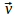is the velocity vector field that describes the velocity of the fluid at each point then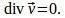This equation follows from the continuity equation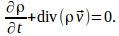First write the continuity equation as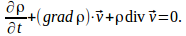Since the fluid is incompressinble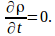There is still the possibility that the fluid can has different densities at different points, but if the fluid is incompressible a fluid element cannot change in density as it moves so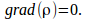Hence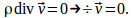If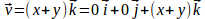then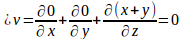and the fluid is incompressible.